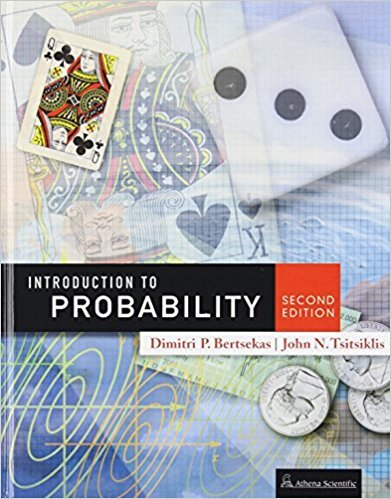×
×

Textbooks / Statistics / Introduction to Probability, 2

# Introduction to Probability, 2nd Edition - Solutions by Chapter## Full solutions for Introduction to Probability, | 2nd Edition

ISBN: 9781886529236Introduction to Probability, | 2nd Edition - Solutions by Chapter

Solutions by Chapter
4 5 0 236 Reviews
##### ISBN: 9781886529236

Introduction to Probability, was written by and is associated to the ISBN: 9781886529236. This expansive textbook survival guide covers the following chapters: 9. The full step-by-step solution to problem in Introduction to Probability, were answered by , our top Statistics solution expert on 01/09/18, 07:43PM. Since problems from 9 chapters in Introduction to Probability, have been answered, more than 5774 students have viewed full step-by-step answer. This textbook survival guide was created for the textbook: Introduction to Probability,, edition: 2.

Key Statistics Terms and definitions covered in this textbook
• 2 k factorial experiment.

A full factorial experiment with k factors and all factors tested at only two levels (settings) each.

• a-error (or a-risk)

In hypothesis testing, an error incurred by failing to reject a null hypothesis when it is actually false (also called a type II error).

• Acceptance region

In hypothesis testing, a region in the sample space of the test statistic such that if the test statistic falls within it, the null hypothesis cannot be rejected. This terminology is used because rejection of H0 is always a strong conclusion and acceptance of H0 is generally a weak conclusion

• Additivity property of x 2

If two independent random variables X1 and X2 are distributed as chi-square with v1 and v2 degrees of freedom, respectively, Y = + X X 1 2 is a chi-square random variable with u = + v v 1 2 degrees of freedom. This generalizes to any number of independent chi-square random variables.

• Alias

In a fractional factorial experiment when certain factor effects cannot be estimated uniquely, they are said to be aliased.

• Bivariate distribution

The joint probability distribution of two random variables.

• Completely randomized design (or experiment)

A type of experimental design in which the treatments or design factors are assigned to the experimental units in a random manner. In designed experiments, a completely randomized design results from running all of the treatment combinations in random order.

• Conditional probability

The probability of an event given that the random experiment produces an outcome in another event.

• Confounding

When a factorial experiment is run in blocks and the blocks are too small to contain a complete replicate of the experiment, one can run a fraction of the replicate in each block, but this results in losing information on some effects. These effects are linked with or confounded with the blocks. In general, when two factors are varied such that their individual effects cannot be determined separately, their effects are said to be confounded.

• Critical value(s)

The value of a statistic corresponding to a stated signiicance level as determined from the sampling distribution. For example, if PZ z PZ ( )( .) . ? =? = 0 025 . 1 96 0 025, then z0 025 . = 1 9. 6 is the critical value of z at the 0.025 level of signiicance. Crossed factors. Another name for factors that are arranged in a factorial experiment.

• Degrees of freedom.

The number of independent comparisons that can be made among the elements of a sample. The term is analogous to the number of degrees of freedom for an object in a dynamic system, which is the number of independent coordinates required to determine the motion of the object.

• Discrete uniform random variable

A discrete random variable with a inite range and constant probability mass function.

• Eficiency

A concept in parameter estimation that uses the variances of different estimators; essentially, an estimator is more eficient than another estimator if it has smaller variance. When estimators are biased, the concept requires modiication.

• Error propagation

An analysis of how the variance of the random variable that represents that output of a system depends on the variances of the inputs. A formula exists when the output is a linear function of the inputs and the formula is simpliied if the inputs are assumed to be independent.

• Event

A subset of a sample space.

• Extra sum of squares method

A method used in regression analysis to conduct a hypothesis test for the additional contribution of one or more variables to a model.

• Finite population correction factor

A term in the formula for the variance of a hypergeometric random variable.

• Fractional factorial experiment

A type of factorial experiment in which not all possible treatment combinations are run. This is usually done to reduce the size of an experiment with several factors.

• Gamma random variable

A random variable that generalizes an Erlang random variable to noninteger values of the parameter r

• Geometric random variable

A discrete random variable that is the number of Bernoulli trials until a success occurs.

×